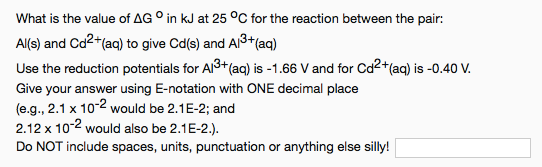# What is the value of ΔG° in kJ at 25°C for the reaction between the pair: Al(s) and Cd2+(aq) to give Cd(s) and Al3+(aq) Use the reduction potentials for Al3+(aq) is -1.66 V and for Cd2+(aq) is -0.40 V. Give your answer using E-notation with ONE decimal place. (e.g., 2 x 10^-2 would be 2E-2; and 2.12 x 10^-2 would also be 2E-2.)